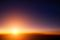cancel
Showing results for
Did you mean:Helper I

## CROSSFILTER to use one dimension measure with multiple fact analyses

Hi all,

I have a rather complex problem, at least I think it is. I do have a sample file further down, if you'd like to play around.

Assume a star schema model, in a very simple form with two fact tables and one product dimension. Within the product dimension, there's a stock value column. I created a measure [ProductStockValue] to be able to show the product stock value in various combinations, e.g. either in combination with Orders or with Invoices - always depending on the current question to analyze.

My approach was to use the CROSSFILTER Funktion, which would be easy if it was just one fact table. Is there a way, to somehow query what fact table I am using and use a corresponding version of the CROSSFILTER function?
I have tried the following code snippets for my measure, for clear understanding please see the following sample file: PBIX FILE

ProductStockValue_Test1 = IF(SELECTEDVALUE ( 'Order'[ProductID] ) = SELECTEDVALUE ( 'Product'[ProductID] )
,CALCULATE(SUM('Product'[StockValue]) ,CROSSFILTER('Product'[ProductID],'Order'[ProductID],BOTH))
,IF ( SELECTEDVALUE ( 'Invoice'[ProductID] ) = SELECTEDVALUE ( 'Product'[ProductID] )
,CALCULATE(SUM('Product'[StockValue]) ,CROSSFILTER('Product'[ProductID],'Invoice'[ProductID],BOTH))
,CALCULATE(SUM('Product'[StockValue]) )) )

ProductStockValue_Test2 = IF(ISFILTERED('Order'[ProductID])=TRUE()
,CALCULATE(SUM('Product'[StockValue]),CROSSFILTER('Product'[ProductID],'Order'[ProductID],BOTH))
,IF(ISFILTERED('Invoice'[ProductID])=TRUE()
,CALCULATE(SUM('Product'[StockValue]),CROSSFILTER('Product'[ProductID],'Invoice'[ProductID],BOTH))))

Best regards,

Kathrin

1 ACCEPTED SOLUTIONSuper User

``````ProductStockValue_AO =
SWITCH (
TRUE (),
ISFILTERED ( 'Order' ), CALCULATE ( SUM ( 'Product'[StockValue] ), 'Order' ),
ISFILTERED ( Invoice ), CALCULATE ( SUM ( 'Product'[StockValue] ), Invoice ),
SUM ( 'Product'[StockValue] )
)``````

Note that if you update your second formula slightly, it sort of works:

``````ProductStockValue_Test2 =
IF (
ISFILTERED ( 'Order'[OrderID] ) = TRUE (),
CALCULATE (
SUM ( 'Product'[StockValue] ),
CROSSFILTER ( 'Product'[ProductID], 'Order'[ProductID], BOTH )
),
IF (
ISFILTERED ( 'Invoice'[InvoiceID] ) = TRUE (),
CALCULATE (
SUM ( 'Product'[StockValue] ),
CROSSFILTER ( 'Product'[ProductID], 'Invoice'[ProductID], BOTH )
)
)
)``````

It works since you have OrderID or InvoiceID in the table but wouldn't if you used a name instead of an ID. ISCROSSFILTERED checks for indirect filters but that would be too broad since Product filters both other tables and you has a filter on it.

2 REPLIES 2Helper I

Hi Alexis,

works like a charm, thank you so much!

BR,

KathrinSuper User

``````ProductStockValue_AO =
SWITCH (
TRUE (),
ISFILTERED ( 'Order' ), CALCULATE ( SUM ( 'Product'[StockValue] ), 'Order' ),
ISFILTERED ( Invoice ), CALCULATE ( SUM ( 'Product'[StockValue] ), Invoice ),
SUM ( 'Product'[StockValue] )
)``````

Note that if you update your second formula slightly, it sort of works:

``````ProductStockValue_Test2 =
IF (
ISFILTERED ( 'Order'[OrderID] ) = TRUE (),
CALCULATE (
SUM ( 'Product'[StockValue] ),
CROSSFILTER ( 'Product'[ProductID], 'Order'[ProductID], BOTH )
),
IF (
ISFILTERED ( 'Invoice'[InvoiceID] ) = TRUE (),
CALCULATE (
SUM ( 'Product'[StockValue] ),
CROSSFILTER ( 'Product'[ProductID], 'Invoice'[ProductID], BOTH )
)
)
)``````

It works since you have OrderID or InvoiceID in the table but wouldn't if you used a name instead of an ID. ISCROSSFILTERED checks for indirect filters but that would be too broad since Product filters both other tables and you has a filter on it.